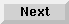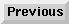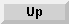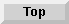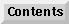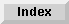2.8 Miscellaneous features

# 2.8.2 Destructuring

Destructuring allows you to bind a set of variables to a corresponding set of values anywhere that you can normally bind a value to a single variable. During`loop` expansion, each variable in the variable list is matched with the values in the values list. If there are more variables in the variable list than there are values in the values list, the remaining variables are given a value of`nil`. If there are more values than variables listed, the extra values are discarded.

Suppose you want to assign values from a list to the variables`a`,`b`, and`c`. You could use one`for` clause to bind the variable`numlist` to the`car` of the specified expression, and then you could use another`for` clause to bind the variables`a`,`b`, and`c` sequentially.

```;; Collect values by using FOR constructs.
> (loop for numlist in '((1 2 4.0) (5 6 8.3) (8 9 10.4))
for a integer = (first numlist)
and for b integer = (second numlist)
and for c float = (third numlist)
collect (list c b a))
((4.0 2 1) (8.3 6 5) (10.4 9 8))

```
Destructuring makes this process easier by allowing the variables to be bound in parallel in each loop iteration. You can declare data types by using a list of type-spec arguments. If all the types are the same, you can use a shorthand destructuring syntax, as the second example illustrates.

```;; Destructuring simplifies the process.
> (loop for (a b c) (integer integer float) in
'((1 2 4.0) (5 6 8.3) (8 9 10.4))
collect (list c b a))
((4.0 2 1) (8.3 6 5) (10.4 9 8))

;; If all the types are the same, this way is even simpler.
> (loop for (a b c) float in
'((1.0 2.0 4.0) (5.0 6.0 8.3) (8.0 9.0 10.4))
collect (list c b a))
((4.0 2.0 1.0) (8.3 6.0 5.0) (10.4 9.0 8.0))

```
If you use destructuring to declare or initialize a number of groups of variables into types, you can use the loop keyword`and` to simplify the process further.

```;; Initialize and declare variables in parallel
;; by using the AND construct.
> (loop with (a b) float = '(1.0 2.0)
and (c d) integer = '(3 4)
and (e f)
return (list a b c d e f))
(1.0 2.0 3 4 NIL NIL)

```
If`nil` is used in a destructuring list, no variable is provided for its place.

```> (loop for (a nil b) = '(1 2 3)
do (return (list a b)))
(1 3)

```
Note that nonstandard lists can specify destructuring.

```> (loop for (x . y) = '(1 . 2)
do (return y))
2

> (loop for ((a . b) (c . d))
((float . float) (integer . integer))
in '(((1.2 . 2.4) (3 . 4)) ((3.4 . 4.6) (5 . 6)))
collect (list a b c d))
((1.2 2.4 3 4) (3.4 4.6 5 6))

```

The Loop Facility - 9 SEP 1996Generated with Harlequin WebMaker